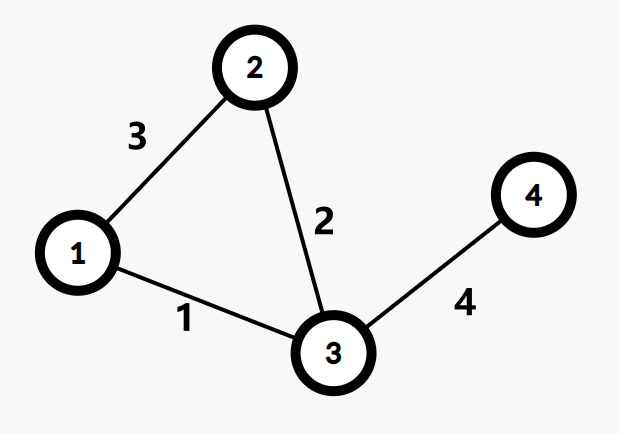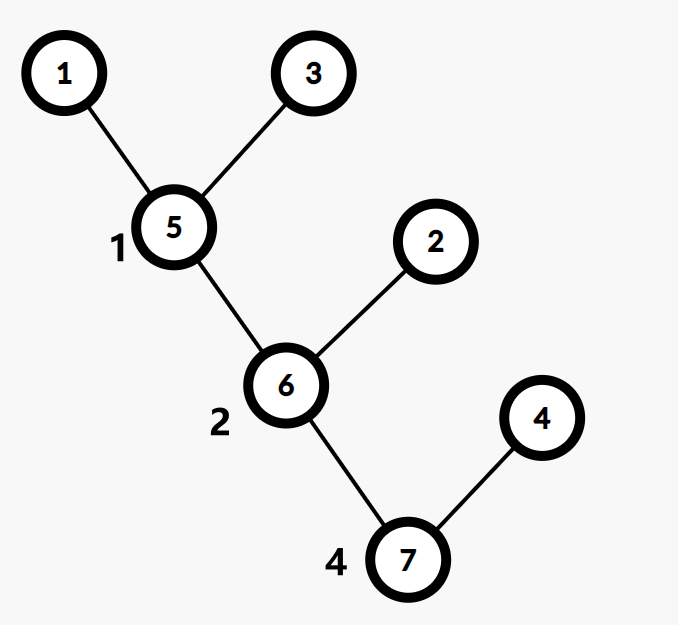# 最小生成树

1. 生成子图
2. 生成树

## Kruskal 算法¶

Kruskal 算法是一种常见并且好写的最小生成树算法，由 Kruskal 发明。该算法的基本思想是从小到大加入边，是个贪心算法。

### 实现¶## Prim 算法¶

Prim 算法是另一种常见并且好写的最小生成树算法。该算法的基本思想是从一个结点开始，不断加点（而不是 Kruskal 算法的加边）。

### 实现¶Fib 堆：

## Boruvka 算法¶

1. 定义 为我们当前找到的最小生成森林的边。在算法执行过程中，我们逐步向 加边，定义 连通块 表示一个点集 ，且这个点集中的任意两个点 中的边构成的子图上是连通的（互相可达）。
2. 定义一个连通块的 最小边 为它连向其它连通块的边中权值最小的那一条。

1. 计算每个点分别属于哪个连通块。将每个连通块都设为“没有最小边”。
2. 遍历每条边 ，如果 不在同一个连通块，就用这条边的边权分别更新 所在连通块的最小边。
3. 如果所有连通块都没有最小边，退出程序，此时的 就是原图最小生成森林的边集。否则，将每个有最小边的连通块的最小边加入 ，返回第一步。## 最小生成树的唯一性¶

  1 2 3 4 5 6 7 8 9 10 11 12 13 14 15 16 17 18 19 20 21 22 23 24 25 26 27 28 29 30 31 32 33 34 35 36 37 38 39 40 41 42 43 44 45 46 47 48 49 50 51 52 53 54 55 56 57 58  #include #include struct Edge { int x, y, z; }; int f; Edge a; int cmp(const Edge& a, const Edge& b) { return a.z < b.z; } int find(int x) { return f[x] == x ? x : f[x] = find(f[x]); } int main() { int t; scanf("%d", &t); while (t--) { int n, m; scanf("%d%d", &n, &m); for (int i = 1; i <= n; i++) f[i] = i; for (int i = 1; i <= m; i++) scanf("%d%d%d", &a[i].x, &a[i].y, &a[i].z); std::sort(a + 1, a + m + 1, cmp); // 先排序 int num = 0, ans = 0, tail = 0, sum1 = 0, sum2 = 0; bool flag = 1; for (int i = 1; i <= m + 1; i++) { // 再并查集加边 if (i > tail) { if (sum1 != sum2) { flag = 0; break; } sum1 = 0; for (int j = i; j <= m + 1; j++) { if (a[j].z != a[i].z) { tail = j - 1; break; } if (find(a[j].x) != find(a[j].y)) ++sum1; } sum2 = 0; } if (i > m) break; int x = find(a[i].x); int y = find(a[i].y); if (x != y && num != n - 1) { sum2++; num++; f[x] = f[y]; ans += a[i].z; } } if (flag) printf("%d\n", ans); else printf("Not Unique!\n"); } return 0; } 

## 次小生成树¶

### 非严格次小生成树¶

#### 求解方法¶

• 求出无向图的最小生成树 ，设其权值和为
• 遍历每条未被选中的边 ，找到 路径上边权最大的一条边 ，则在 中以 替换 ，可得一棵权值和为 的生成树 .
• 对所有替换得到的答案 取最小值即可

### 严格次小生成树¶

#### 代码¶

  1 2 3 4 5 6 7 8 9 10 11 12 13 14 15 16 17 18 19 20 21 22 23 24 25 26 27 28 29 30 31 32 33 34 35 36 37 38 39 40 41 42 43 44 45 46 47 48 49 50 51 52 53 54 55 56 57 58 59 60 61 62 63 64 65 66 67 68 69 70 71 72 73 74 75 76 77 78 79 80 81 82 83 84 85 86 87 88 89 90 91 92 93 94 95 96 97 98 99 100 101 102 103 104 105 106 107 108 109 110 111 112 113 114 115 116 117 118 119 120 121 122 123 124 125 126 127 128 129 130 131 132 133 134 135 136 137 138 139 140 141 142 143 144 145 146 147 148 149 150 151 152 153 154 155 #include #include const int INF = 0x3fffffff; const long long INF64 = 0x3fffffffffffffffLL; struct Edge { int u, v, val; bool operator<(const Edge &other) const { return val < other.val; } }; Edge e; bool used; int n, m; long long sum; class Tr { private: struct Edge { int to, nxt, val; } e; int cnt, head; int pnt; int dpth; // 到祖先的路径上边权最大的边 int maxx; // 到祖先的路径上边权次大的边，若不存在则为 -INF int minn; public: void addedge(int u, int v, int val) { e[++cnt] = (Edge){v, head[u], val}; head[u] = cnt; } void insedge(int u, int v, int val) { addedge(u, v, val); addedge(v, u, val); } void dfs(int now, int fa) { dpth[now] = dpth[fa] + 1; pnt[now] = fa; minn[now] = -INF; for (int i = 1; (1 << i) <= dpth[now]; i++) { pnt[now][i] = pnt[pnt[now][i - 1]][i - 1]; int kk = {maxx[now][i - 1], maxx[pnt[now][i - 1]][i - 1], minn[now][i - 1], minn[pnt[now][i - 1]][i - 1]}; // 从四个值中取得最大值 std::sort(kk, kk + 4); maxx[now][i] = kk; // 取得严格次大值 int ptr = 2; while (ptr >= 0 && kk[ptr] == kk) ptr--; minn[now][i] = (ptr == -1 ? -INF : kk[ptr]); } for (int i = head[now]; i; i = e[i].nxt) { if (e[i].to != fa) { maxx[e[i].to] = e[i].val; dfs(e[i].to, now); } } } int lca(int a, int b) { if (dpth[a] < dpth[b]) std::swap(a, b); for (int i = 21; i >= 0; i--) if (dpth[pnt[a][i]] >= dpth[b]) a = pnt[a][i]; if (a == b) return a; for (int i = 21; i >= 0; i--) { if (pnt[a][i] != pnt[b][i]) { a = pnt[a][i]; b = pnt[b][i]; } } return pnt[a]; } int query(int a, int b, int val) { int res = -INF; for (int i = 21; i >= 0; i--) { if (dpth[pnt[a][i]] >= dpth[b]) { if (val != maxx[a][i]) res = std::max(res, maxx[a][i]); else res = std::max(res, minn[a][i]); a = pnt[a][i]; } } return res; } } tr; int fa; int find(int x) { return fa[x] == x ? x : fa[x] = find(fa[x]); } void Kruskal() { int tot = 0; std::sort(e + 1, e + m + 1); for (int i = 1; i <= n; i++) fa[i] = i; for (int i = 1; i <= m; i++) { int a = find(e[i].u); int b = find(e[i].v); if (a != b) { fa[a] = b; tot++; tr.insedge(e[i].u, e[i].v, e[i].val); sum += e[i].val; used[i] = 1; } if (tot == n - 1) break; } } int main() { std::ios::sync_with_stdio(0); std::cin.tie(0); std::cout.tie(0); std::cin >> n >> m; for (int i = 1; i <= m; i++) { int u, v, val; std::cin >> u >> v >> val; e[i] = (Edge){u, v, val}; } Kruskal(); long long ans = INF64; tr.dfs(1, 0); for (int i = 1; i <= m; i++) { if (!used[i]) { int _lca = tr.lca(e[i].u, e[i].v); // 找到路径上不等于 e[i].val 的最大边权 long long tmpa = tr.query(e[i].u, _lca, e[i].val); long long tmpb = tr.query(e[i].v, _lca, e[i].val); // 这样的边可能不存在，只在这样的边存在时更新答案 if (std::max(tmpa, tmpb) > -INF) ans = std::min(ans, sum - std::max(tmpa, tmpb) + e[i].val); } } // 次小生成树不存在时输出 -1 std::cout << (ans == INF64 ? -1 : ans) << '\n'; return 0; } 

## 瓶颈生成树¶

### 例题¶

POJ 2395 Out of Hay

## 最小瓶颈路¶

### 性质¶1 到 4 的最小瓶颈路显然有以下两条：1-2-3-4。1-3-4。

## Kruskal 重构树¶

### 定义¶### 性质¶

「LOJ 137」最小瓶颈路 加强版
  1 2 3 4 5 6 7 8 9 10 11 12 13 14 15 16 17 18 19 20 21 22 23 24 25 26 27 28 29 30 31 32 33 34 35 36 37 38 39 40 41 42 43 44 45 46 47 48 49 50 51 52 53 54 55 56 57 58 59 60 61 62 63 64 65 66 67 68 69 70 71 72 73 74 75 76 77 78 79 80 81 82 83 84 85 86 87 88 89 90 91 92 93 94 95 96 97 98 99 100 101 102 103 104 105 106 107 108 109 110 111 112 113 114 115 116 117 118 119 120 121 122 123 124 125 126 127 128 129 130 131 132 133 134 135 136 137  #include using namespace std; const int MAX_VAL_RANGE = 280010; int n, m, log2Values[MAX_VAL_RANGE + 1]; namespace TR { struct Edge { int to, nxt, val; } e; int cnt, head; void addedge(int u, int v, int val = 0) { e[++cnt] = (Edge){v, head[u], val}; head[u] = cnt; } int val; namespace LCA { int sec, cnt; int pos; int dpth; void dfs(int now, int fa) { dpth[now] = dpth[fa] + 1; sec[++cnt] = now; pos[now] = cnt; for (int i = head[now]; i; i = e[i].nxt) { if (fa != e[i].to) { dfs(e[i].to, now); sec[++cnt] = now; } } } int dp; void init() { dfs(2 * n - 1, 0); for (int i = 1; i <= 4 * n; i++) { dp[i] = sec[i]; } for (int j = 1; j <= 19; j++) { for (int i = 1; i + (1 << j) - 1 <= 4 * n; i++) { dp[i][j] = dpth[dp[i][j - 1]] < dpth[dp[i + (1 << (j - 1))][j - 1]] ? dp[i][j - 1] : dp[i + (1 << (j - 1))][j - 1]; } } } int lca(int x, int y) { int l = pos[x], r = pos[y]; if (l > r) { swap(l, r); } int k = log2Values[r - l + 1]; return dpth[dp[l][k]] < dpth[dp[r - (1 << k) + 1][k]] ? dp[l][k] : dp[r - (1 << k) + 1][k]; } } // namespace LCA } // namespace TR using TR::addedge; namespace GR { struct Edge { int u, v, val; bool operator<(const Edge &other) const { return val < other.val; } } e; int fa; int find(int x) { return fa[x] == 0 ? x : fa[x] = find(fa[x]); } void kruskal() { // 最小生成树 int tot = 0, cnt = n; sort(e + 1, e + m + 1); for (int i = 1; i <= m; i++) { int fau = find(e[i].u), fav = find(e[i].v); if (fau != fav) { cnt++; fa[fau] = fa[fav] = cnt; addedge(fau, cnt); addedge(cnt, fau); addedge(fav, cnt); addedge(cnt, fav); TR::val[cnt] = e[i].val; tot++; } if (tot == n - 1) { break; } } } } // namespace GR int ans; int A, B, C, P; inline int rnd() { return A = (A * B + C) % P; } void initLog2() { for (int i = 2; i <= MAX_VAL_RANGE; i++) { log2Values[i] = log2Values[i >> 1] + 1; } } int main() { initLog2(); // 预处理 cin >> n >> m; for (int i = 1; i <= m; i++) { int u, v, val; cin >> u >> v >> val; GR::e[i] = (GR::Edge){u, v, val}; } GR::kruskal(); TR::LCA::init(); int Q; cin >> Q; cin >> A >> B >> C >> P; while (Q--) { int u = rnd() % n + 1, v = rnd() % n + 1; ans += TR::val[TR::LCA::lca(u, v)]; ans %= 1000000007; } cout << ans; return 0; } 
NOI 2018 归程